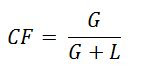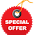## Friday, March 2, 2012

### Working Capital Inventory - The Critical Fractile Method

Up to this point, we have explored the inventory elements that comprise the Cash Conversion Cycle in a static fashion.
In Working Capital – Finance and Inventory we looked at how inventory can impact our risk and cost of capital.
In Working Capital – Economic Order Quantity we looked at a common formula to optimize our inventory ordering or production activity, with an exploration of its strengths, weaknesses, and hidden “traps”.
Today we turn to the first method that incorporates probability into the mix. This has the great advantage of reflecting the uncertainty involved in operating a business, a fact that has been ignored or assumed away in methods up to this point.
In other words, it’s a little more realistic, isn’t it?

The Critical Fractile Model
The Critical Fractile model, in one sense, is an incremental method of viewing inventory ordering or production. By “incremental” we mean we can use it to look at things from a “one step at a time” viewpoint - if we are at 10, we look at 9 and 11. If we are at 100, we look at 99 and 101.
To take a numerical example, let’s say we operate a hot dog cart on a street corner sidewalk of your favorite city (mine is Chicago, of course, though once I visit Hong Kong next month, who knows!).
Our hot dogs cost us ²1 each (for new readers, ² is the symbol for Treasury Café Monetary Units, or TCMU’s). We sell our hot dogs for ²3 each, so for each sale our margin is ²2. Currently we buy 50 hot dogs per day - some days we sell out and some days we don’t. As our pushcart requires cooked hot dogs, they are not reusable so all left over items are discarded.Figure A
In an effort to improve our performance, we have tasked our highly skilled finance and treasury analysts to crunch some Big Data, and from that analysis have determined that for our street corner the hot dog demand averages 50 with a standard deviation of 20 and is in the form of a normal distribution. Given this distribution, Figure A shows the probability a hot dog is needed at all possible values between 0 and 100.
Earlier we noted we have been stocking 50 hot dogs. Is this optimal, or would we be better off stocking only 49 instead? When using the Critical Fractile method, we compare the incremental probability of gain and loss. At issue currently is the 50th hot dog.Figure B
Because we know the demand probabilities, we can calculate our “Expected Value” for carrying the 50th hot dog. The term Expected Value comes from probability theory, and in this case can be represented by the equation in Figure B.
For the 50th hot dog in our inventory, we have a 50% chance of selling it (pG =.5, G = ²2) and a 50% chance of not selling it (pL =.5, L = ²1). Therefore, by our equation in Figure B, our expected value from carrying the 50th hot dog is ²0.50. Since this is a positive contribution, stocking the 50th hot dog makes sense.
Perhaps then it makes sense to stock 51 hot dogs? Based on our normal curve, there is a 52% probability this will not be sold, and a 48% probability that it will. Given these probabilities and our gain and loss figures of ²2 and ²1, using the equation in Figure B we can calculate that our expected value from carrying the 51st hot dog is ²0.44. Since this is a positive number, we will maximize our business by carrying this additional unit.

From Incremental to CumulativeFigure C
In Figure C, the orange line shows the incremental contribution for each hot dog stocked (units of measurement are on the left vertical axis). Notice the orange line starts on the left-hand side of the graph near ²2. This is the same amount we gain on the sale of a hot dog. Since the probability of selling 1 hot dog is well over 99%, it makes sense that the line is almost ²2 on the left-hand side.
On the right hand side the orange line is negative. If we stocked the 100th hot dog, we would almost never sell it (less than 1% of the time), so it is very close to the cost of holding inventory we cannot sell: ²1.
The dark brown line on the graph is our cumulative earnings (it’s measurement is the right axis). On the very left hand side, the brown line starts around ²2 (Why? Because if we only had 1 hot dog, and almost always sold it, we would make almost ²2 per day). It goes to ²10 pretty quick, because even the probability of selling the 5th hot dog is over 98%, so somewhere between 5 and 6 hot dogs per day the brown line reflects ²10 in earnings.

The Critical Fractile – and Grand Finale
In Figure C, our cumulative earnings, reflected by the brown line, is hump shaped. The top of that hump is our maximum earnings potential given the combination of our gains, losses and probability distribution.Figure D
Notice that the peak earnings coincide to the point on the graph where the orange line, our incremental gain or loss based on probability, goes below 0. This makes sense – if each additional hot dog has an expected loss, then our earnings will be lowered by these additional losses as we add them. Calculus lovers will note that the incremental earnings for each unit is a derivative measure of our total earnings function.
Figure D shows the Critical Fractile equation, which is a ratio of the incremental gain vs. the combined incremental gain and loss. In our case, since the gain is ²2, and the gain and loss combined is ²2 + ²1 = ²3, then the Critical Fractile equation equals .6666~.
Figure E shows what we can do with this equation. The graph is our cumulative probability of a hot dog not being needed (i.e. a mirror image of what we looked at earlier in Figure A).Figure E
The orange line is the Critical Fractile: 0.6666~. When the Critical Fractile line touches our cumulative probability, the brown line then traces down to the number of hot dogs, a number between 58 and 59.
You will notice that this is the same point in Figure C where our incremental line passes from above 0 to below, and is the point of our maximum earnings!
If we know our gain on a sale, and our loss on no sale, along with a probability distribution we are in a position to determine our optimal inventory level merely be following the process leading to Figure D:
a)      Calculate the Critical Fractile per the equation in Figure D,
b)      Determine where this intersects our probability distribution,
c)      Determine the associated inventory level at the intersection point.

Other Considerations
The analysis above did not consider a number of factors that some will encounter. In some cases, our inventory is not perishable, and thus an item that is not sold may be held for another day. In this case we would need to modify our Loss figure to reflect the time value of money and inventory carrying cost associated with holding that for an additional period.
In other cases, a lost sale does not result in a permanent loss, but might only be delayed due to a back-order type process. We can again accommodate this in the gain and loss calculations prior to running our Critical Fractile process.
Finally, sometimes a lost sale results in a greater than one sale loss. Wireless companies often think in these regards. If a customer has a contract, there is some probability they will renew when it is up, and when that contract is up there is some probability they will renew again. Thus, losing a sale today also involves losing some sales next period and the period thereafter. This concept is known as the Customer Lifetime Value, and this would be incorporated into our loss figure for purposes of the Critical Fractile process.

Key Takeaways
The Critical Fractile method is an inventory and production quantity method which incorporates variability in demand, and therefore is somewhat more representative of situations a business faces than more static calculations.
Questions
·         What is your experience in using the Critical Fractile Method?

Add to the discussion with your thoughts, comments, questions and feedback! Please share Treasury Café with others. Thank you!

1.Absolutely fascinating. I could never have imagined that such a relatively sophisticated and extensive calculus could have gone into an example as simple as a hot dog cart. It really makes one thing about the type of calculations involved in this area for a Fortune 500 company!

1.Farm Land,

While we might be able to use calculus for something as simple as a hot dog cart, the converse is also sometimes (emphasis on sometimes) true, we may use something simple to deal with the incredibly complex.

Yet, as you suggest, the method presented above often can be insufficient for some F500 items.

Thank you for adding to the discussion!

2.David,

I like this article, mainly because it applies theory and techniques developed for managing the physical supply chain to the finnacial supply chain. It has always been my belief that finance managers can learn much more from their colleagues in logistics and production.
We need to be aware that reality is a bit more resillient and certainly not alway adhearing to the Gauss Curve. But having said that around an optimum the probablity curves are always horizontal.

1.Bas Rebel,

Being a fan of Taleeb, I will be the first to agree with you that use of the Gaussian curve is often used in inappropriate situations.

It is my understanding that the Critical Fractile can be used with non-normal distributions, if nothing more than replicating Figure E above, draw a line from the fractile until it intersects the Cumulative Distribution line, and then draw down to the quantity.

I also agree with you halfways about learning - finance folks can learn a lot from "operating" folks, but the reverse is also true.

In my experience there are a lot of operating decisions that are not necessarily financially sound due to financial factors not being considered, but most finance folks then approach this by dealing with the operating folks from an arrogant, "ivory-tower" perspective, which destroys any chance that productive teamwork will occur.

Part of my hope for the recent working capital focus in this blog was to use some models and concepts that can bridge the two and achieve "common-ground" type thinking, as any one involved in an organization will need if the hope is to avoid siloism.

Thank you for adding to the discussion!

3.No woman gets an orgasm from shining the kitchen floor. Hey, i am looking for an online sexual partner ;) Click on my boobs if you are interested (. )( .)

4.